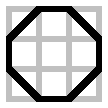### Problem Statement

You have a rectangle with corners at (0, 0) and (m, n). You would like to place a convex polygon inside it such that the polygon's vertices are at integer coordinates. The vertices may lie anywhere inside the rectangle or on its boundary.

You are given m and n, the dimensions of the rectangle. Return the maximal number of vertices contained by the convex polygon.

### Definition

 Class: ConvexHull Method: intHull Parameters: int, int Returns: int Method signature: int intHull(int m, int n) (be sure your method is public)

### Constraints

-m and n will be between 3 and 200, inclusive.

### Examples

0)

 `3` `3`
`Returns: 8`1)

 `3` `50`
`Returns: 8`
2)

 `4` `4`
`Returns: 9`
3)

 `4` `5`
`Returns: 10`4)

 `50` `200`
`Returns: 74`

#### Problem url:

http://www.topcoder.com/stat?c=problem_statement&pm=4787

#### Problem stats url:

http://www.topcoder.com/tc?module=ProblemDetail&rd=8068&pm=4787

Vedensky

#### Testers:

PabloGilberto , brett1479 , Olexiy

#### Problem categories:

Brute Force, Dynamic Programming